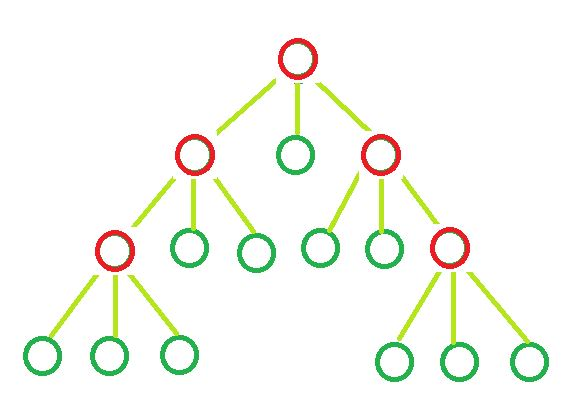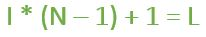Related Articles

# Determine the count of Leaf nodes in an N-ary tree

• Difficulty Level : Medium
• Last Updated : 13 Apr, 2021

Given the value of ‘N’ and ‘I’. Here,represents the number of internal nodes present in an N-ary tree and every node of the N-ary can either havechilds or zero child. The task is to determine the number of Leaf nodes in n-ary tree.

Examples:

Input : N = 3, I = 5
Output : Leaf nodes = 11Input : N = 10, I = 10
Output : leaf nodes = 91

Formula:where,
I = Number of Internal nodes.
L = Leaf Nodes.
and, N = Number of children each node can have.

Derivation: The tree is an N-ary tree. Assume it has T total nodes, which is the sum of internal nodes (I) and leaf nodes (L). A tree with T total nodes will have (T – 1) edges or branches.
In other words, since the tree is an N-ary tree, each internal node will have N branches contributing a total of N*I internal branches. Therefore we have the following relations from the above explanations,

• N * I = T – 1
• L + I = T

From the above two equations, we can say that L = (N – 1) * I + 1.

Below is the implementation of the above approach:

## C++

 `// CPP program to find number``// of leaf nodes` `#include ``using` `namespace` `std;` `// Function to calculate``// leaf nodes in n-ary tree``int` `calcNodes(``int` `N, ``int` `I)``{``    ``int` `result = 0;` `    ``result = I * (N - 1) + 1;` `    ``return` `result;``}` `// Driver code``int` `main()``{``    ``int` `N = 5, I = 2;` `    ``cout << ``"Leaf nodes = "` `<< calcNodes(N, I);` `    ``return` `0;``}`

## Java

 `// Java program to find number``// of leaf nodes` `class` `GfG``{` `// Function to calculate``// leaf nodes in n-ary tree``static` `int` `calcNodes(``int` `N, ``int` `I)``{``    ``int` `result = ``0``;` `    ``result = I * (N - ``1``) + ``1``;` `    ``return` `result;``}` `// Driver code``public` `static` `void` `main(String[] args)``{``    ``int` `N = ``5``, I = ``2``;` `    ``System.out.println(``"Leaf nodes = "` `+``                        ``calcNodes(N, I));``}``}` `// This code is contributed by Prerna Saini`

## Python3

 `# Python3 program to find number``# of leaf nodes` `# Function to calculate``# leaf nodes in n-ary tree``def` `calcNodes(N, I):``    ``result ``=` `0` `    ``result ``=` `I ``*` `(N ``-` `1``) ``+` `1` `    ``return` `result` `# Driver Code``if` `__name__ ``=``=` `'__main__'``:``    ``N ``=` `5``    ``I ``=` `2` `    ``print``(``"Leaf nodes = "``,``           ``calcNodes(N, I))` `# This code is contributed``# by SHUBHAMSINGH10`

## C#

 `// C# program to find number``// of leaf nodes``using` `System;` `class` `GFG``{` `// Function to calculate``// leaf nodes in n-ary tree``static` `int` `calcNodes(``int` `N, ``int` `I)``{``    ``int` `result = 0;` `    ``result = I * (N - 1) + 1;` `    ``return` `result;``}` `// Driver code``public` `static` `void` `Main()``{``    ``int` `N = 5, I = 2;` `    ``Console.Write(``"Leaf nodes = "` `+``                  ``calcNodes(N, I));``}``}` `// This code is contributed``// by Akanksha Rai`

## PHP

 ``

## Javascript

 ``
Output:
`Leaf nodes = 9`

Attention reader! Don’t stop learning now. Get hold of all the important DSA concepts with the DSA Self Paced Course at a student-friendly price and become industry ready.  To complete your preparation from learning a language to DS Algo and many more,  please refer Complete Interview Preparation Course.

In case you wish to attend live classes with experts, please refer DSA Live Classes for Working Professionals and Competitive Programming Live for Students.

My Personal Notes arrow_drop_up